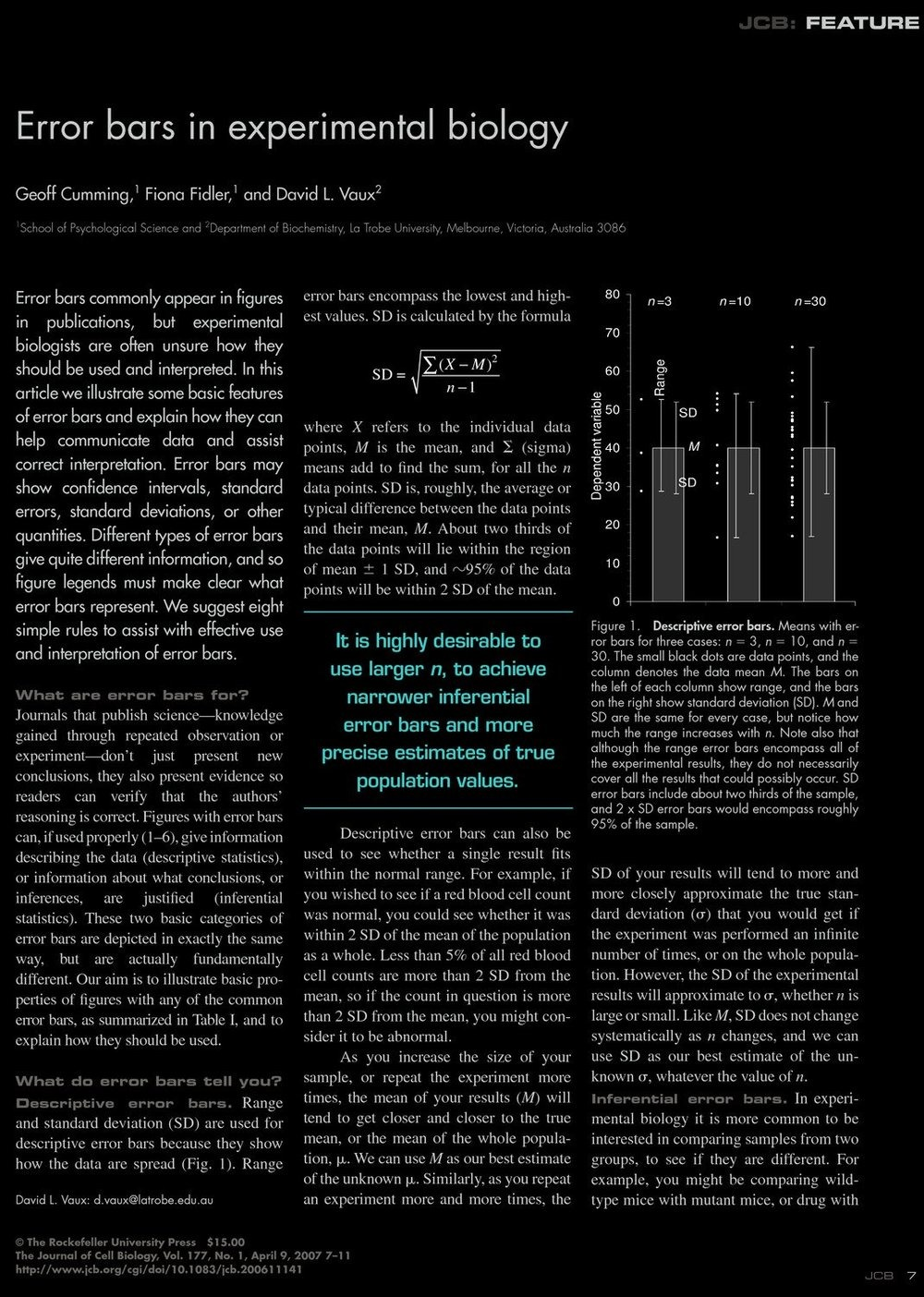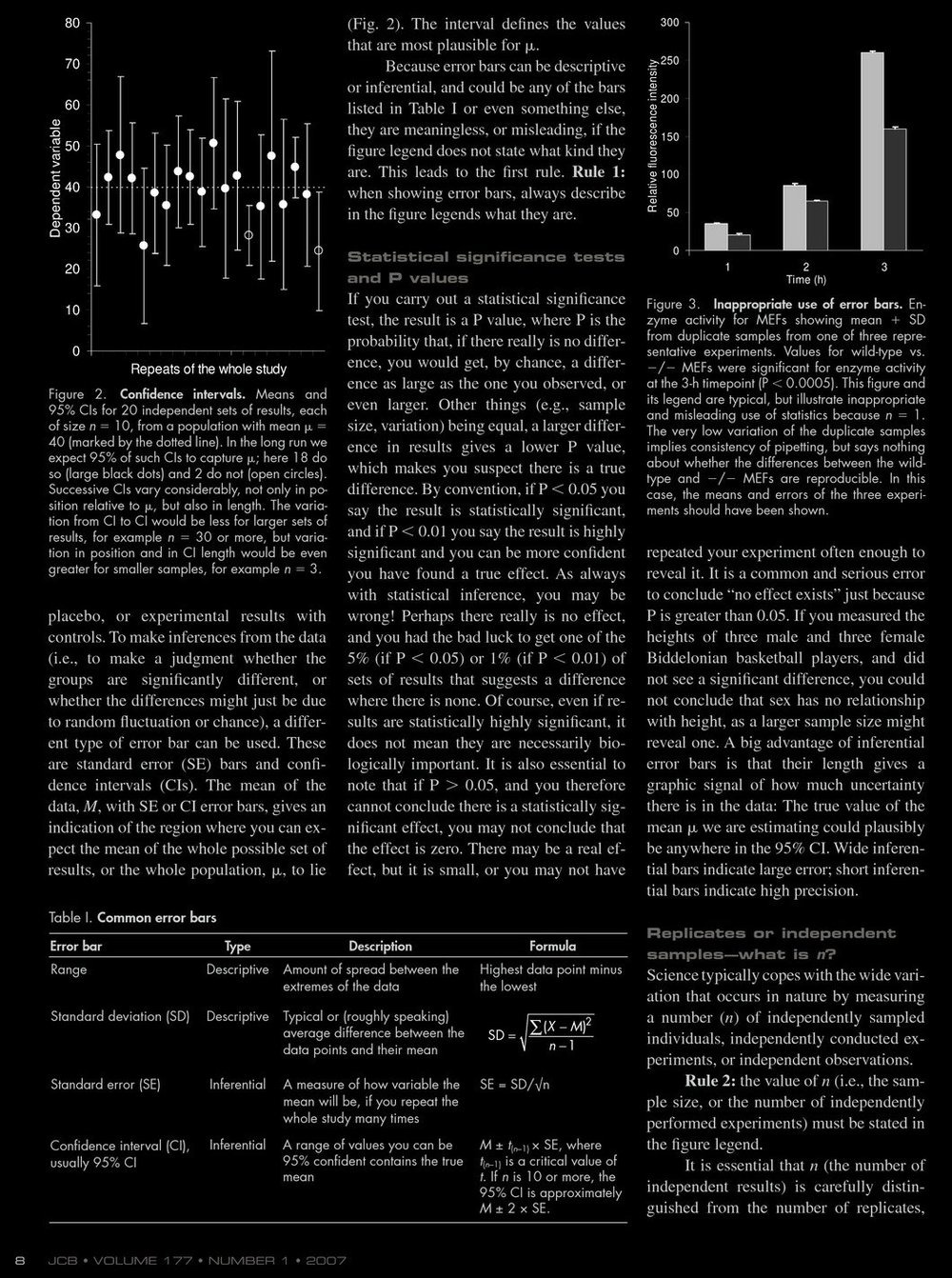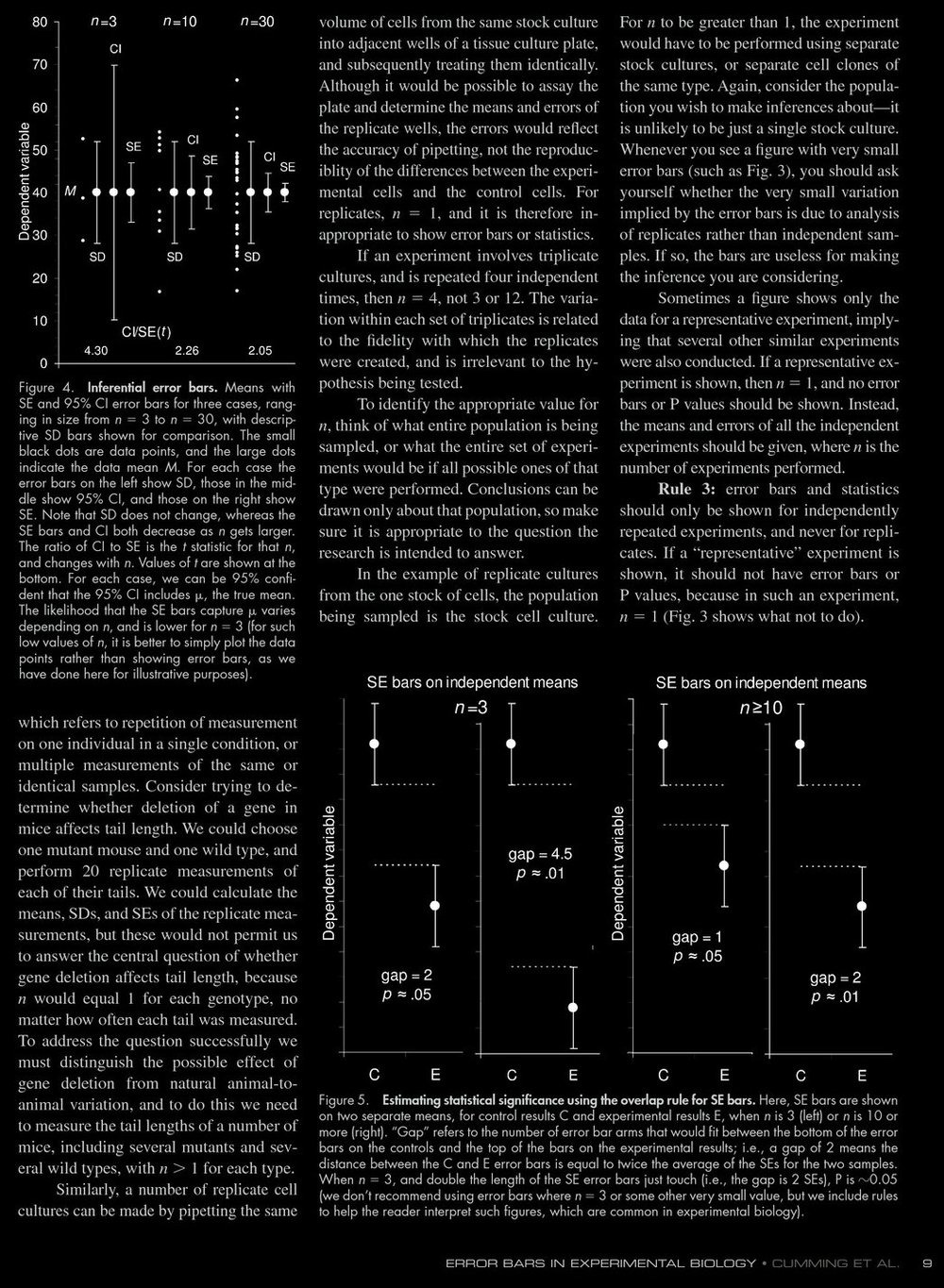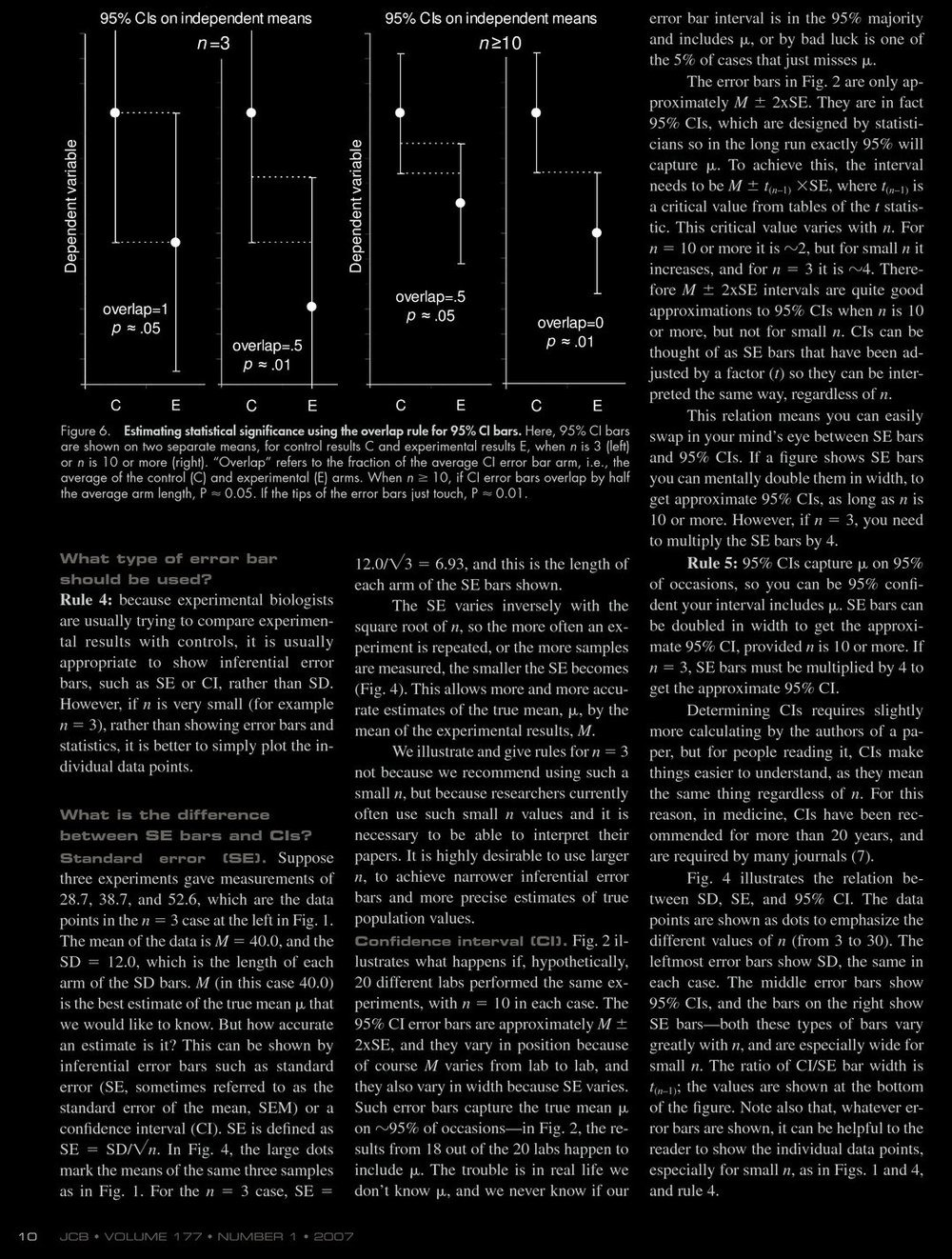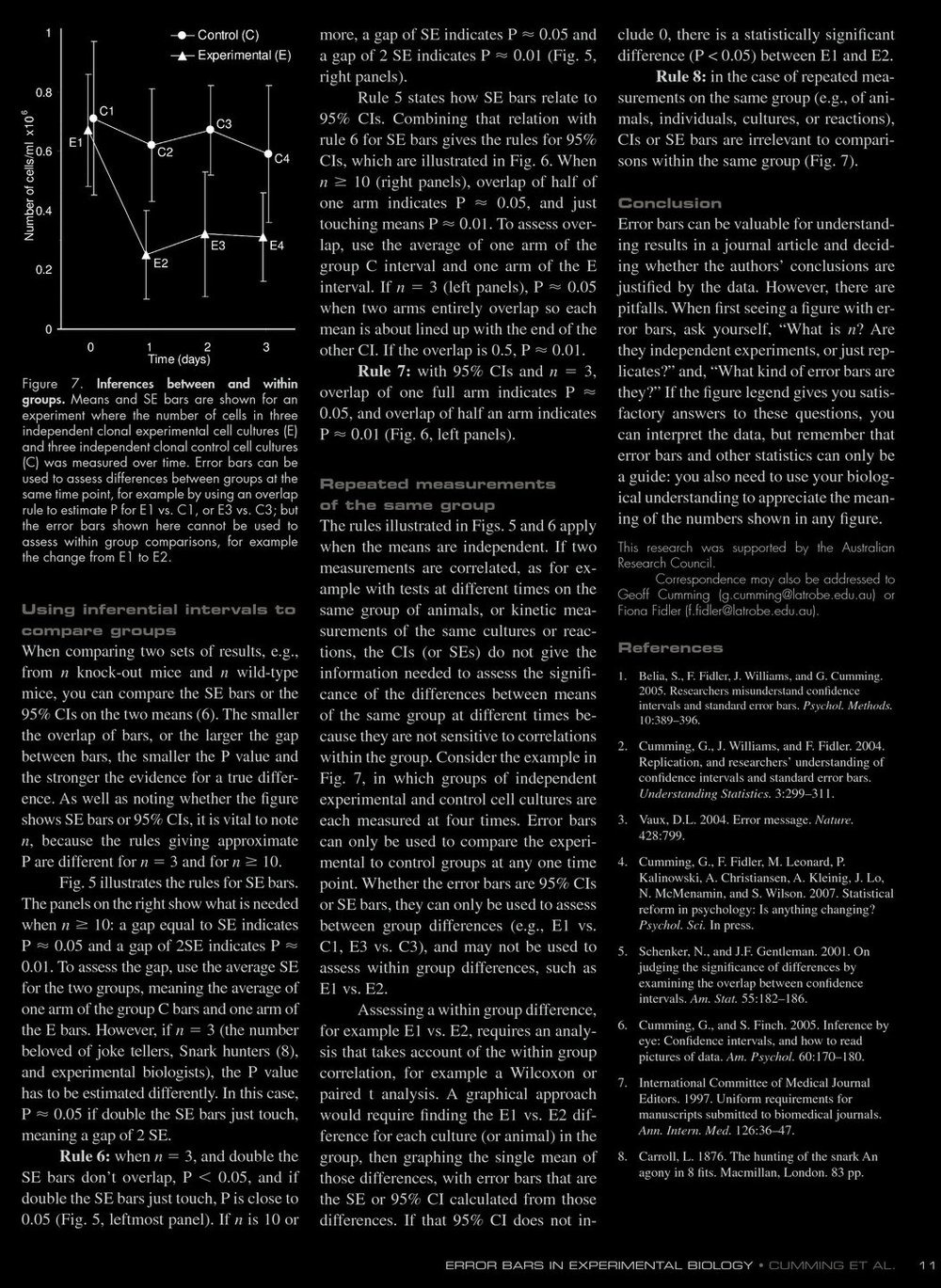# On the use of Error bars

The following article of Geoff Cumming,Fiona Fidler,1 and David L. Vaux: Error bars in experimental biology, Journal of Cell biology, 177, 1, 2007 7-11, has momentarily the second highest readership in Mendeley: it addresses the meaning of error bars in scientific papers. It mentions the following rules:

• Rule 1: When showing error bars, describe what they are.

• Rule 2: Indicate the number of experiments.
• Rule 3: Use error bars and statistics only for independently repeated experiments, not replicates.
• Rule 4: Show the standard error SE=SD/n1/2 or confidence interval CI rather than standard deviation SD.
• Rule 5: SE can be doubled in width to get 95 percent CI if n is 10 or more.
• Rule 6: A gap of SE indicates P value 0.05 ands statistic significance if n is 10 or more.
• Rule 7: with 95 CI and n=3, overlap of one full arm indicates P=0.05
• Rule 8: For repeated measurements of same groups, CI, SE are irrelevant for comparisons within the same group.
Important terms which appear and are explained: The standard deviation SD and the standard error SE (which is SD divided by the square root of the number of experiments). An other important quantity in scientific experiments is the P-value: Assume we have a random variable X over some probability space and we measure X=c. The question is, whether this experiment is significant or not assuming a null-hypothesis (which stands for the setup of our probability space). The P-value of the experiment is defined as
```  p = P[ X > c]
```
By convention (note this is arbitrary and therefore a bit controversial), one calls p smaller than 0.05 a statistically significant result and a P-value smaller than 0.01 a highly significant result. For example, if you see 10 times head when throwing a coin 30 times. What is the P value? If X is the number of heads, then P[X smaller or equal to 10] = F, here computed with Mathematica
```f=CDF[BinomialDistribution[30,0.5]]; f
```
is 0.0493. This is considered statistically significant. With this assumptions we would considered it a significant test that the null hypothesis (the coin is fair and different coin experiments are independent) is rejected. However, if see 11 heads, then the P-value is slightly larger than 0.1 and the test is not significant. You see how easy it is to cheat here: Just repeat your coin flipping experiment a lot until you reach an instance with a statistically significant deviation result. This will eventually happen. Suppress the other experiments as "test trials" and publish the result.